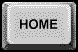AUTOMATED MANUFACTURING

Course Objectives:
1. Introduce principles, methods, and hardware/software tools used in modern computerized design and manufacturing of discrete parts.
2. Acquire practical experience in computer-aided design and manufacturing through a series of laboratory exercises (see ADMS Laboratory)
3. Understand the main components involved in automated manufacturing, so as to be able to select an area of academic and professional specialization.

Course Outline:
·  Introduction to geometric modeling and related techniques for computer-aided design, process planning, and manufacturing: approximating curves and surfaces, motion planning.
· Introduction to basic concepts of probability theory for Statistical Process Control (SPC): quality control, control charts, Taguchi methods
· Discrete-event control of manufacturing systems: Programmable Logic Control (PLC)
· Introduction to systems and control theory: modeling and control of dynamic processes, applications to manufacturing process control and robotics.
· Supervisory control of manufacturing systems: discrete-event models, inventory control, scheduling.

LABORATORY SESSIONS:
1. Designing a part to be manufactured.
2. Computer-Aided Design (CAD): designing a part using the SmartCAM software
3. CNC machining: introduction and basic operation of a CNC milling machine
4. Computer-Aided Process Planning (CAPP): creating a process model for turning using the SmartCAM software
5. Process planning and CNC machining of a rotational part: manufacturing a part on a lathe
6. Programmable Logic Controllers (PLC): introduction, basic operation, and programming of a PLC
7. Robotics: introduction and basic operation of a robotic manipulator
8. Robot control: controlling a robotic manipulator through the ACL language
9. Vision systems for inspection of parts
10. Statistical Process Control (SPC): introduction to basic techniques for inspection and quality control
11. Computer Integrated Manufacturing (CIM): introduction to communication networking in a manufacturing environment
12. Computer Simulation and supervisory control of manufacturing systems: task sequencing and scheduling

STATISTICS AND QUALITY ENGINEERING

Course Objectives:
1. Introduce principles of probability and statistics including events, Bayes theorem, random variables, functions of random variables, sampling distributions, and parameter estimation.

2. Learn about the main concepts of quality engineering: Acceptance Sampling, Real Time Quality Control, and the Taguchi method for product quality improvement.

Course Outline:
·  Probabilistic modeling; statistical/probabilistic thinking, reasoning and decision making; data analysis, communicating statistical information.

·  Treatment of Data: Frequency distributions, Descriptive statistics

·  Probability Theory basics

·  Probability Distribution and Density: Random variables and probability distributions, special distribution and density functions, mean and variance, functions of random variables, vectors of random variables and joint density and distribution

·  Sampling Distributions

·  Statistical Inference: Estimation and confidence intervals, hypothesis testing

·  Statistical methods for Quality Control and Improvement: Acceptance sampling, Control charts, Design of Experiments

PROBABILITY THEORY IN ELECTRICAL AND COMPUTER ENGINEERING

Course Objectives:
1. Develop a solid foundation in the concepts of probability theory.

2. Learn fundamental probabilistic modeling and analysis techniques so you can use them in Electrical and Computer Engineering applications.

3. Learn basic techniques for managing and processing data and apply them to basic estimation and hypothesis testing problems.

Course Outline:
- Foundations of Probability Theory

- Discrete and continuous random variables

- Pairs of random variables: joint cumulative distribution and density functions, marginal probability density functions, functions of random variables, expectation, covariance, correlation, conditioning

- Sums of random variables: sample averages, moment generating functions, inequalities, Central Limit Theorem

- Parameter estimations: point estimation, interval estimation

- Hypothesis testing